Next: Exercises Up: Dispersive Waves Previous: Ship Wakes

# Capillary Waves

Water in contact with air actually possesses a finite surface tension,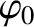(Haynes and Lide 2011b), which allows there to be a small pressure discontinuity across a free surface that is curved. In fact,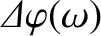(987)

(Batchelor 2000). Here,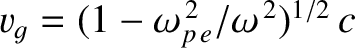is the radius of curvature of the surface. Thus, in the presence of surface tension, the boundary condition (938) takes the modified form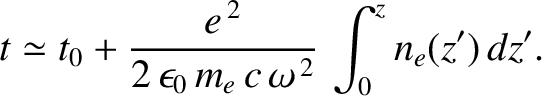(988)

which reduces to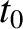(989)

This boundary condition can be combined with the solution (943), in the deep water limit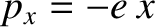, to give the modified deep water dispersion relation (see Exercise 21)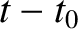(990)

Hence, the phase velocity of the waves takes the form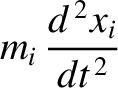(991)

and the ratio of the group velocity to the phase velocity can be shown to be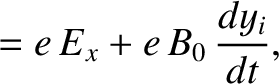(992)

We conclude that the phase velocity of surface water waves attains a minimum value of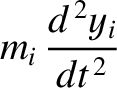when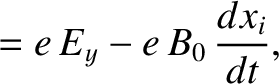, which corresponds to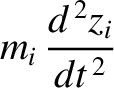. The group velocity equals the phase velocity at this wavelength. For long wavelength waves (i.e.,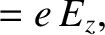), gravity dominates surface tension, the phase velocity scales as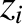, and the group velocity is half the phase velocity. As we have already mentioned, this type of wave is known as a gravity wave. On the other hand, for short wavelength waves (i.e.,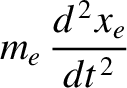), surface tension dominates gravity, the phase velocity scales as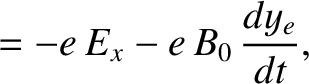, and the group velocity is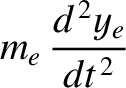times the phase velocity. This type of wave is known as a capillary wave. The fact that the phase velocity and the group velocity both attain minimum values whenmeans that when a wave disturbance containing a wide spectrum of wavelengths, such as might be generated by throwing a rock into the water, travels across the surface of a lake, and reaches the shore, the short and long wavelength components of the disturbance generally arrive before the components of intermediate wavelength.Next: Exercises Up: Dispersive Waves Previous: Ship Wakes
Richard Fitzpatrick 2013-04-08